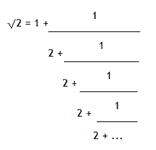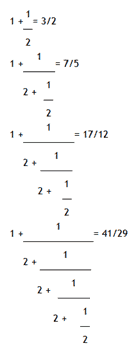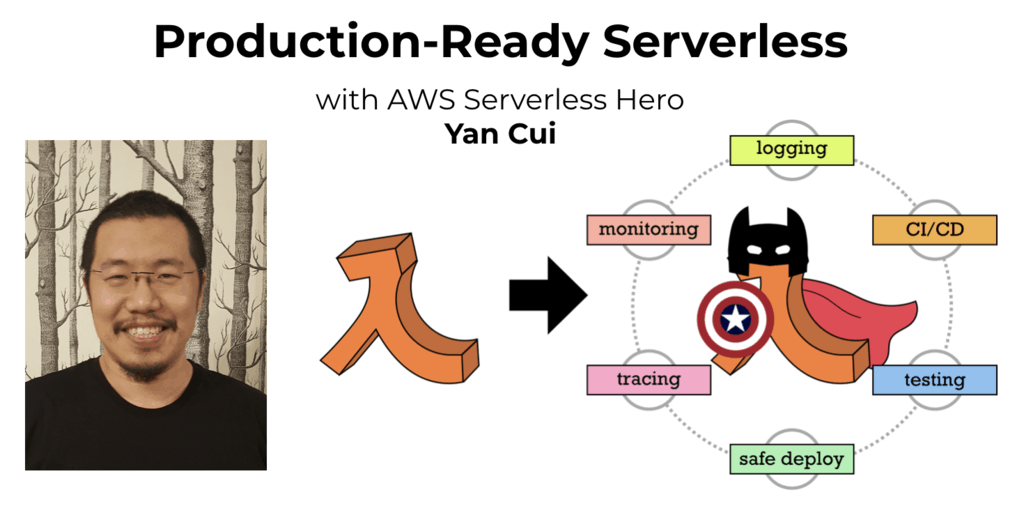# Project Euler – Problem 65 Solution

Check out my new course Learn you some Lambda best practice for great good! and learn the best practices for performance, cost, security, resilience, observability and scalability.

#### Problem

The square root of 2 can be written as an infinite continued fraction.The infinite continued fraction can be written, ?2 = [1;(2)], (2) indicates that 2 repeats ad infinitum. In a similar way, ?23 = [4;(1,3,1,8)].

It turns out that the sequence of partial values of continued fractions for square roots provide the best rational approximations. Let us consider the convergents for ?2.Hence the sequence of the first ten convergents for ?2 are:

1, 3/2, 7/5, 17/12, 41/29, 99/70, 239/169, 577/408, 1393/985, 3363/2378, …

What is most surprising is that the important mathematical constant,

e = [2; 1,2,1, 1,4,1, 1,6,1 , … , 1,2k,1, …].

The first ten terms in the sequence of convergents for e are:

2, 3, 8/3, 11/4, 19/7, 87/32, 106/39, 193/71, 1264/465, 1457/536, …

The sum of digits in the numerator of the 10th convergent is 1+4+5+7=17.

Find the sum of digits in the numerator of the 100th convergent of the continued fraction for e.

#### Solution

If you look at the convergents of ?2 and the numerators in the convergents of e, you’ll see a pattern emerging:

1    +    2    *   1    =    3

2    +    3    *   2    =    8

3    +    8    *   1    =    11

8    +   11   *   1    =    19

11  +   19   *   4    =    87

If you look at the sequence of numerators in the convergents of e ( 2, 3, 8, 11, … ) and the sequence of numbers in the convergents of ?2 ( 1, 2, 1, 1, 4, 1, … ), given the current numerator ( n ) and the previous numerator ( n-1 ) in the sequence and the corresponding number in the convergents of ?2 ( i )the next numerator ( n+1 ) can be calculated using the formula:

( n+1) = ( n-1 ) + n * i

Once we have this formula to work with, the rest is simple, the solution runs in 7 milliseconds on my machine.Hi, I’m Yan. I’m an AWS Serverless Hero and the author of Production-Ready Serverless.

I specialise in rapidly transitioning teams to serverless and building production-ready services on AWS.

Are you struggling with serverless or need guidance on best practices? Do you want someone to review your architecture and help you avoid costly mistakes down the line? Whatever the case, I’m here to help.Check out my new course, Learn you some Lambda best practice for great good! In this course, you will learn best practices for working with AWS Lambda in terms of performance, cost, security, scalability, resilience and observability. Enrol now and enjoy a special preorder price of £9.99 (~\$13).Are you working with Serverless and looking for expert training to level-up your skills? Or are you looking for a solid foundation to start from? Look no further, register for my Production-Ready Serverless workshop to learn how to build production-grade Serverless applications!